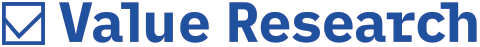# Growth vs Dividend Reinvestment

##### What is the basic difference between a growth option and dividend reinvestment? Will there be any difference in the overall return if a person invested Rs 1,00,000 in growth option and the same amount in reinvestment option? -Ajit Bhardwaj

What is the basic difference between a growth option and dividend reinvestment? Will there be any difference in the overall return if a person invested Rs 1,00,000 in growth option and the same amount in reinvestment option?
—Ajit Bhardwaj

The difference for investment in the growth and dividend option comes solely from the difference in their tax status. Equity funds and debt funds attract different taxes and this will affect the returns. For equity funds, the dividend distribution tax is nil.

However, in this year's budget, long-term capital gains tax has been abolished, while short term capital gains has been reduced to 10 per cent. This implies that for an investment made for more than one year, there will be no tax on the gains.

In case the investment is redeemed within a year though, there may be an incidence of a capital gains tax. This will happen if, after taking into consideration the extra units allotted under the dividend reinvestment option, one is still left with some gains. This gain would constitute capital gains for him and would attract a short-term capital gains tax of 10 per cent.

Taking your case of an investment of Rs 1,00,000, let us assume that the same amount has been invested in dividend reinvestment option and growth option. Assuming that the fund gives an eight per cent return, the total investment would grow to Rs 1,08,000. If Rs 5,000 is given as dividend, then the investor would have realised Rs 3,000 as capital appreciation. Now, if an investor decides to redeem the investment within an year, a short-term capital gains tax would be applicable only as dividends are tax-free. At a rate of 10 per cent (plus surcharge of 2 per cent), the investor would have to pay Rs 306 as tax. This would reduce his returns to Rs 7,694.

For long-term, assuming that the return is the same at Rs 8,000, the investor will get Rs 8,000 irrespective of whether he invests in dividend reinvestment option or the growth option.

For debt mutual funds, dividends are still subject to a dividend distribution tax of 12.5 per cent (plus surcharges) for retail investors and a 20 per cent (plus surcharge) dividend distribution tax for corporates. There has been no change in the capital gains structure. The gains still attract both short-term capital gains and long-term capital gains.

The implication is as follows. Say, as an individual investor, your investment of Rs 1,00,000 generates a return of 5 per cent. This would amount to Rs 5,000. If Rs 4,000 out of this is distributed as dividend, after the levy of dividend distribution tax of 12.5 per cent (plus surcharge), the amount that the investor gets would come to Rs 3537.67.

After dividend is distributed, the investor is left with capital appreciation of Rs 1,000. If the investor redeems his money within a year, there would be a short-term capital gains tax on this. Assuming the tax rate to be 30 per cent (plus surcharge), the investor would have to pay a Rs 306 tax and the amount of capital gain left with him would be Rs 694. Together with the dividend that the investor receives, his total return after taxes would be Rs 4,231.67.

If the investor redeems after a year, he would face a long-term capital gains tax. At a rate of 10 per cent (plus surcharge of 2 per cent) this would amount to Rs 102. The amount of capital gains after taxes would get reduced to Rs 898. Taking the dividend into consideration, his returns come to get Rs 4,435.67.

Thus, if the investor withdraws from a debt fund in the short-term, for a return of Rs 5,000, he gets Rs 4,231.67 if he has invested in the dividend distribution model and Rs 3,470 if he has invested in the growth model. In the long-term, he gets Rs 4,435.67 from the dividend distribution model and Rs 4,490 from the growth model.